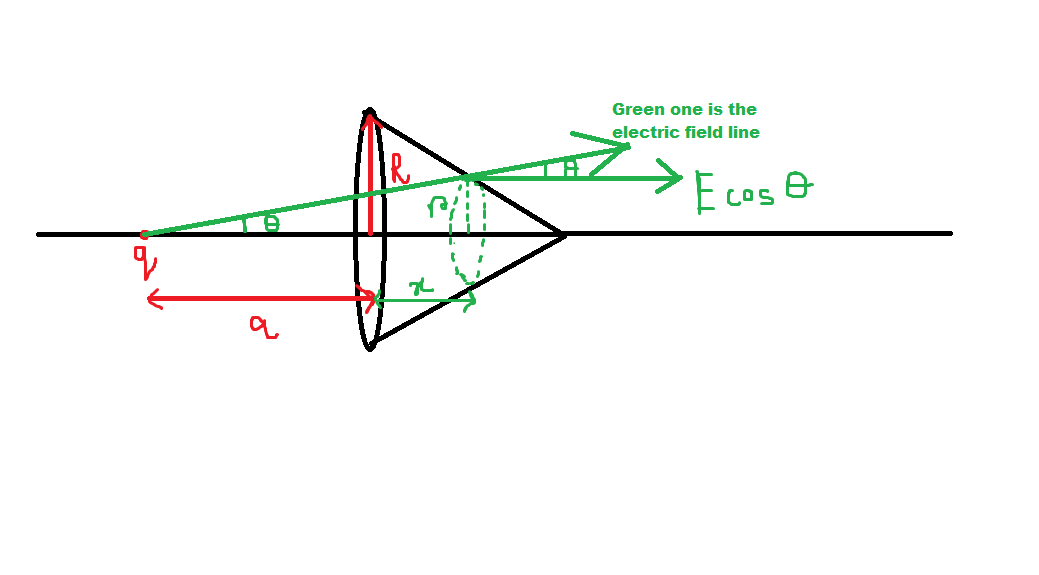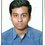# Sorry, But another Doubt! :P (Original)

Hey everyone! Sorry for disturbing again,I again have a doubt!

It will be very helpful, If you help me clearing it out.

Now lets jump into the question!

QUESTION

A cone is placed and a charge is placed at a distance of a m from the base of the cone as shown in the figure.For our ease, Lets take $a=1m$ , $R=1m$ , $h=1m$ ( height of the cone).

## What we need to find is the flux due to the charge q through the cone!

MY SOLUTION

Here it goes, First of all, lets find the radius of the small disc we took,

Using Proportionality in triangles, we have

$\dfrac{R}{h} = \dfrac{r}{h-x}$

$\implies r=1-x$. This is the radius of the disc.

So the elemental area = $2 \pi (1-x) (-dx)$.

Now Our flux is like this,

$\phi = \displaystyle{\int_{S} E \cos \theta \times dS}$

$\implies \phi= \displaystyle{\int_{0}^{1} \dfrac{q(1+x) \times -2 \pi (1-x) dx}{4 \pi \epsilon_{0} \times (2(1+x^2))^{3/2}}}$

$\implies \phi = \displaystyle{\int_{0}^{1} \dfrac{q ( x^2-1) dx}{4\sqrt{2} \epsilon_{0} (1+x^2)^{3/2}}}$

After evaluating the integral, we get

$\implies \phi = \dfrac{q}{4 \sqrt{2} \epsilon_{0}} \dfrac{\sqrt{2} \times arsinh(1)-2}{\sqrt{2}}$

$\boxed{\implies \phi = \dfrac{q}{4 \sqrt{2} \epsilon_{0}} \dfrac{\sqrt{2} \times arsinh(1)-2}{\sqrt{2}}}$

But unfortunately our $\phi$ comes out to be $-ve$.

Please help!!!

Thanks in Advance!Note by Md Zuhair
3 years, 2 months ago

This discussion board is a place to discuss our Daily Challenges and the math and science related to those challenges. Explanations are more than just a solution — they should explain the steps and thinking strategies that you used to obtain the solution. Comments should further the discussion of math and science.

When posting on Brilliant:

• Use the emojis to react to an explanation, whether you're congratulating a job well done , or just really confused .
• Ask specific questions about the challenge or the steps in somebody's explanation. Well-posed questions can add a lot to the discussion, but posting "I don't understand!" doesn't help anyone.
• Try to contribute something new to the discussion, whether it is an extension, generalization or other idea related to the challenge.
• Stay on topic — we're all here to learn more about math and science, not to hear about your favorite get-rich-quick scheme or current world events.

MarkdownAppears as
*italics* or _italics_ italics
**bold** or __bold__ bold
- bulleted- list
• bulleted
• list
1. numbered2. list
1. numbered
2. list
Note: you must add a full line of space before and after lists for them to show up correctly
paragraph 1paragraph 2

paragraph 1

paragraph 2

[example link](https://brilliant.org)example link
> This is a quote
This is a quote
    # I indented these lines
# 4 spaces, and now they show
# up as a code block.

print "hello world"
# I indented these lines
# 4 spaces, and now they show
# up as a code block.

print "hello world"
MathAppears as
Remember to wrap math in $$ ... $$ or $ ... $ to ensure proper formatting.
2 \times 3 $2 \times 3$
2^{34} $2^{34}$
a_{i-1} $a_{i-1}$
\frac{2}{3} $\frac{2}{3}$
\sqrt{2} $\sqrt{2}$
\sum_{i=1}^3 $\sum_{i=1}^3$
\sin \theta $\sin \theta$
\boxed{123} $\boxed{123}$

## Comments

Sort by:

Top Newest

I guess you guys will try helping me finding the correct relation of $\phi$ as I am getting -ve.

Thanks in Advance! I will be eagerly waiting for your response!

- 3 years, 2 months ago

Log in to reply

Courtesy of Gauss' Theorem. the flux through the curved surface area of the cone is equal to the flux through its circular base $C$. Thus \begin{aligned} \Phi & = \; \iint_C \mathbf{E} \cdot d\mathbf{S} \; = \; \frac{q}{4\pi \varepsilon_0} \iint_C \frac{\mathbf{r} \cdot d\mathbf{S}}{r^3} \; = \; \frac{q}{4\pi \varepsilon_0}\int_0^R \frac{a}{(a^2 + u^2)^{\frac32}}\,2\pi u\,du \\ & = \; \frac{qa}{2\varepsilon_0}\Big[-(a^2 + u^2)^{-\frac12}\Big]_{u=0}^R \; = \; \frac{qa}{2\varepsilon_0}\left(\frac{1}{a} - \frac{1}{\sqrt{a^2 + R^2}}\right) \end{aligned} and with the given parameters we have $\Phi \; = \; \frac{q}{2\varepsilon_0}\left(1 - \tfrac{1}{\sqrt{2}}\right)$

You are not calculating $\mathbf{E}\cdot d\mathbf{S}$ correctly. You are using $E \,\cos\theta \,dS$. In other words, you are assuming that $d\mathbf{S}$ is horizontal (which works when integrating across the base $C$), but this is not true when you are trying to integrate over the curved surface area of the cone.

- 3 years, 2 months ago

Log in to reply

But if we are talkimg about the cylinders with same case of placement of charges then we see that they go by my method. Can you plz explain that.. as there also we can just find for base. Thanks.

- 3 years, 2 months ago

Log in to reply

If we had a cylinder, the flux through the curved surface would be the flux through the near disc minus the flux through the far disc (so the total flux out of the cylinder is zero), and we can use my method again.

If you insist on integrating over the curved surface, the normal component of $\mathbf{E}$ would be $E\sin\theta$. You could integrate that over the surface.

- 3 years, 2 months ago

Log in to reply

Oh I see.. So Esin theta dS will make it? But then what will be the dS? The same one?

- 3 years, 2 months ago

Log in to reply

Is the cone solid or hollow?

- 3 years, 2 months ago

Log in to reply

solid...

- 3 years, 2 months ago

Log in to reply

Isn't the total flux through any closed surface due to some charge placed outside the body/surface =0 (Gauss Law)?

- 3 years, 2 months ago

Log in to reply

yeah... but its the total. i want the curved SA flux

- 3 years, 2 months ago

Log in to reply

$dS$ would be the usual area element on a cylinder, yes. I should point out that one of the many benefits of Gauss' Theorem is that is allows us to avoid nasty integrals, and instead integrate over simpler surfaces. You are making life hard for yourself.

- 3 years, 2 months ago

Log in to reply

True that sir. It is more clever by using Gauss Law rather than integrating. Thanks for your response! :D

- 3 years, 2 months ago

Log in to reply

×

Problem Loading...

Note Loading...

Set Loading...